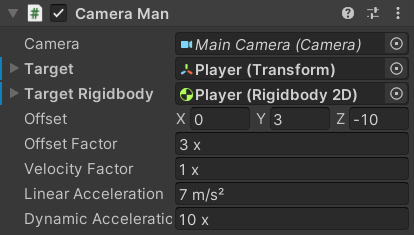﻿ Platformer Game Kit - Camera Man

# Camera Man

The `CameraMan` script is responsible for moving the `Camera` to follow the Player.It has references to the `Camera` as well as the Player's `Transform` and `Rigidbody2D`:

``````public sealed class CameraMan : MonoBehaviour
{
[SerializeField]
[Tooltip("The camera this script is controlling")]
private Camera _Camera;
public ref Camera Camera => ref _Camera;

[SerializeField]
[Tooltip("The object this script is tracking")]
private Transform _Target;
public ref Transform Target => ref _Target;

[SerializeField]
[Tooltip("[Optional] The Rigidbody2D of the Target")]
private Rigidbody2D _TargetRigidbody;
public ref Rigidbody2D TargetRigidbody => ref _TargetRigidbody;
``````

There are also various other fields to determine the way it moves with Units Attributes where appropriate:

``````    [SerializeField]
[Tooltip("The position offset from the target to the camera")]
private Vector3 _Offset;
public ref Vector3 Offset => ref _Offset;

[SerializeField, Multiplier]
[Tooltip("Determines how much the distance from the camera to where it is supposed to be affects its velocity")]
private float _OffsetFactor = 1;
public ref float OffsetFactor => ref _OffsetFactor;

[SerializeField, Multiplier]
[Tooltip("Determines how much the velocity of the Target Rigidbody affects the velocity of the camera")]
private float _VelocityFactor = 1;
public ref float VelocityFactor => ref _VelocityFactor;

[SerializeField, MetersPerSecondPerSecond]
[Tooltip("The flat rate at which the camera accelerates")]
private float _LinearAcceleration = 1;
public ref float LinearAcceleration => ref _LinearAcceleration;

[SerializeField, Multiplier]
[Tooltip("The additional rate at which the camera accelerates in proportion to how far away its velocity is from the desired value")]
private float _DynamicAcceleration = 1;
public ref float DynamicAcceleration => ref _DynamicAcceleration;
``````

The `OnValidate` method ensures that all fields have valid values and if there is no `_Target` it tries to find an object with the `"Player"` tag:

``````#if UNITY_EDITOR
private void OnValidate()
{
gameObject.GetComponentInParentOrChildren(ref _Camera);

if ((_Target == null || _TargetRigidbody == null) && !UnityEditor.EditorUtility.IsPersistent(this))
{
var target = GameObject.FindGameObjectWithTag("Player");
if (target != null)
{
if (_Target == null)
_Target = target.transform;

target.GetComponentInParentOrChildren(ref _TargetRigidbody);
}
}

PlatformerUtilities.NotNegative(ref _VelocityFactor);
PlatformerUtilities.NotNegative(ref _OffsetFactor);
PlatformerUtilities.NotNegative(ref _LinearAcceleration);
PlatformerUtilities.NotNegative(ref _DynamicAcceleration);
``````

It also applies the `_Offset` value to its position so that you can immediately see where it will be when making changes in the scene:

``````        if (_Target != null)
{
if (_Offset == default)
_Offset = transform.position - _Target.position;
else
transform.position = _Target.position + _Offset;
}
}
#endif
``````

Since the `Transform` is used frequently, it is stored in a field on startup to improve performance:

``````    private Transform _Transform;

private void Awake()
{
_Transform = transform;
}
``````

The main movement operations are performed in `LateUpdate`.

It has an `[ExecuteAlways]` attribute and `#if UNITY_EDITOR` block to run in Edit Mode:

• If you modify the position of its `Transform` component, it will update its `_Offset` field accordingly (the opposite of `OnValidate`).
• If the `_Target` is moved, the `CameraMan` will automatically follow it.
``````[ExecuteAlways]
public sealed class CameraMan : MonoBehaviour
{
...

private Vector3 _Velocity;

private void LateUpdate()
{
if (_Target == null)
return;

var position = _Transform.position;
var destination = _Target.position + _Offset;

#if UNITY_EDITOR
if (!UnityEditor.EditorApplication.isPlayingOrWillChangePlaymode)
{
if (UnityEditor.Selection.Contains(gameObject))
{
_Offset = position - _Target.position;
}
else
{
_Transform.position = destination;
}

return;
}
#endif
``````

The `Target Rigidbody` field is optional so it only gets the velocity if it has one:

``````        var hasRigidbody = _TargetRigidbody != null;
var velocity = hasRigidbody ? _TargetRigidbody.velocity : default;
``````

If the position the camera is trying to reach is off-screen, it teleports the camera to it immediately instead of moving all the way over gradually:

``````        var screenPosition = _Camera.WorldToScreenPoint(destination);
if (screenPosition.x < 0 ||
screenPosition.y < 0 ||
screenPosition.x > Screen.width ||
screenPosition.y > Screen.height)
{
_Transform.position = destination;
_Velocity = velocity;
return;
}
``````

That happens if the `Target` is teleported far away or can also happen if they fall for too long.

The desired velocity the camera wants to be moving at is calculated using the `_OffsetFactor` and `_VelocityFactor` fields:

``````        var targetVelocity = (destination - position) * _OffsetFactor;

if (_VelocityFactor > 0)
targetVelocity += (Vector3)(velocity * _VelocityFactor);
``````

Then the current `_Velocity` is moved towards that value according to the `_LinearAcceleration` and `_DynamicAcceleration`:

``````        var deltaTime = Time.deltaTime;

_Velocity = Vector3.MoveTowards(_Velocity, targetVelocity, _LinearAcceleration * deltaTime);
_Velocity = Vector3.LerpUnclamped(_Velocity, targetVelocity, _DynamicAcceleration * deltaTime);
``````

And finally, the `_Velocity` is added to the position and applied to the `Transform:

``````        position += _Velocity * deltaTime;

_Transform.position = position;
}
}
``````